# Planck constant

(diff) ← Older revision | Latest revision (diff) | Newer revision → (diff)
An absolute physical constant, having the dimension of action (energy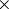time) in the CGS system. Planck's constantis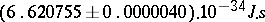(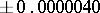is the possible error in the measurement). It was first introduced by M. Planck (1900) in a paper on the radiation of light, in which he suggested that the energyof a photon (an electromagnetic wave) is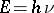, whereis the frequency of the wave. Later, when quantum mechanics arose, Planck's constant was used in the definition of major quantum-mechanical quantities (momentum and energy operators, etc.) and appeared in almost all equations of quantum mechanics.
Planck's constant characterizes in a certain sense the limits of the use of classical mechanics: The laws of quantum mechanics deviate substantially from those of classical mechanics only for physical systems for which the characteristic distances, velocities and masses are such that the corresponding characteristic action is of the same order as. In a formal mathematical treatment this means that the equations of quantum mechanics go over to the corresponding classical equations as.
The constantmay be replaced by the constant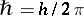, which is also called Planck's constant.